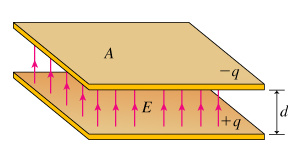1
0
watching
141
views

To understand the meaning of capacitance and ways of calculating capacitance. When a positive charge q is placed on a conductor that is insulated from ground, an electric field emanates from the conductor to ground, and the conductor will have a nonzero potential V relative to ground. If more charge is placed on the conductor, this voltage will increase proportionately. The ratio of charge to voltage is called the capacitance C of this conductor: C=q/V. Capacitance is one of the central concepts in electrostatics, and specially constructed devices called capacitors are essential elements of electronic circuits. In a capacitor, a second conducting surface is placed near the first (they are often called electrodes), and the relevant voltage is the voltage between these two electrodes. This tutorial is designed to help you understand capacitance by assisting you in calculating the capacitance of a parallel-plate capacitor, which consists of two plates each of area A separated by a small distanced with air or vacuum in between. In figuring out the capacitance of this configuration of conductors, it is important to keep in mind that the voltage difference is the line integral of the electric field between the plates.Assume that charge -q is placed on the top plate, and +q is placed on the bottom plate. What is the magnitude of the electric field E between the plates? What is the voltage V between the plates of the capacitor?